### Let D(t) represent the amount of drug in a patient (measured in grams) at time t

Question: Let D(t) represent the amount of drug in a patient (measured in grams) at time t (mea- sured in hour). The initial amount of drug is 90 grams. After 2 hours the amount is 70 grams. Assuming that the amount of drug decays exponentially, address the following problems. (a) Write down a differential equation that best describes the growth of the amount of the drug. (b) Find the general solution to the differential equation in part (a). (c) Use the additional provided information to determine D(t). (d) What is the half-life for D(t)?

Solution:

### How many grams of oxygen are present in 100.0 g Ca(NO3)2 is g.

Question: How many grams of oxygen are present in 100.0 g Ca(NO3)2 is g. Use correct number of significant digits;

Solution: Mol weight of Ca(NO3)2 = 90 + 28 +96
= 164 g/mol
100 g of Ca(NO3)2 = 0.6097 mol of Ca(NO3)2
1 mol of Ca(NO3)2 contains 6 number of oxygen atoms.
so, 0.6097 moles of Ca(NO3)2 contains = 0.6097 * 6 = 3.6582 Now, mole of each ‘O’ atom is 16 g/mol So, 100 g of Ca(NO3)2 contains = 3.6582*16 = 58.5312 g of oxygen.

### A superconductor is a substance that is able to conduct electricity without resistance

Question: A superconductor is a substance that is able to conduct electricity without resistance, a property that is very desirable in the construction of large electromagnets. Metals have this property if cooled to temperatures a few degrees above absolute zero, but this requires the use of expensive liquid helium (boiling point 4 K). Scientists have discovered materials that become superconductors at higher temperatures, but they are ceramics. Their brittle nature has so far prevented them from being made into long wires. A recently discovered compound of magnesium and boron, which consists of 52.9% Mg and 47.1% B, shows special promise as a high-temperature superconductor because it is inexpensive to make and can be fabricated into wire relatively easily. What is the formula of this compound?

Solution:

Let us take 100 g of compound
Then, mass of Mg = 52.9g
and mass of boron = 47.1g
Moles of Mg = Mass/Molar Mass = 52.9g/24.31g/mol
= 2.18 mol
and moles of B = 47.1g/10.81g/mol
= 4.36 mol
So, the molar ratio of Mg and B:
nMg/nB = 2.18 mol/4.36 mol = 1/2
Formula of the compound is MgB2

### Assuming that all the blackbody radiation is emitted at this wavelength

Question: Consider a metal that is being welded

Assuming that all the blackbody radiation is emitted at this wavelength (a crude approximation), how many photons does a square centimeter of the metal emit per second?

Solution: Using stefan-boltzmann law, energy emitted by blackbody
E = σAtT^4
A = 1 cm^2 = 10^-4 m^2
t = 1s
but E =hcn/λ
σ = 5.67 * 10^-8
T = b/λ = 2.898 * 10^-3/λ
n = λσAtT^4/hc
n = 5.6710^-810^-41(2.89810^-3)^4/(6.6310^-34310^8(49010^-9)^3)
n= 1.71*10^22 photons

### Data Communications Networking Chapter 3, Problem 48E

Question: What is the total delay (latency) for a frame of size 5 million bits that is being sent on a link with 10 routers each having a queuing time of 2 μs and a processing time of 1 μs. The length of the link is 2000 Km. The speed of light inside the link is 2 × 108 m/s. The link has a bandwidth of 5 Mbps. Which component of the total delay is dominant? Which one is negligible?

Solution:

Given data:

Distance (length of the link) =2000 Km

=2000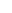103m

Propagation speed=2108m/s

Frame size=5million bits

=5000000bits

The Bandwidth=5Mbps

=5106 bps (1Mbps=106 bps)

Queuing time=102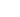(10 routers each having a queuing time of 2)

=20Processing delay=101(10 routers each having a processing time of 1)

=10Total delay (Latency) = Propagation time + Transmission time + Queuing time

+ Processing delay.

Propagation time=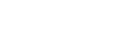=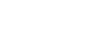= 0.01s

Therefore, Propagation time=0.01s

Transmission time=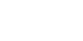=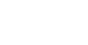= 1s

Therefore, Transmission time=1s

Queuing time=102=10210-6s (1=10-6s)

=2010-6s

= 0.00002s

Therefore, Queuing time=0.00002s

Processing delay=101=1010-6s (1=10-6s)

Therefore, Processing delay=0.000010s

Therefore, Total delay (Latency) =0.01+1+0.000020+0.000010

=1.01003s

### Drag the “orbitals with electrons” box to the energy scale shown.

Question: Drag the “orbitals with electrons” box to the energy scale shown. Your goal in this exercise is to show nitrogen in an sp3-hybridized state which is capable of forming 3 bonds with three other atoms. Fill answer fields from left to right. Place orbitals with two electrons on the left (if any).

Solution: Concepts and reason

An orbital is the distribution of the probability density that is associated with the given electron centered at a given point. If the point is the nucleus of an atom, an atomic orbital is the resultant. If the point is the center of mass of multiple nuclei within a molecule, a molecular orbital is the resultant.

When an atom is required to bond with the other atoms to form a molecule, the valence atomic orbitals with almost similar energies ‘hybridize’ to form hybridized orbitals. These orbitals of identical shapes have identical associated energies.

The number of hybrid orbitals formed is equal to the number of atomic orbital hybridizing. Fundamentals

The hybridization of an atom in a molecule could be correlated with the number of bonds formed by it. This is tabulated as follows:

The sum of the principal quantum number and the azimuthal quantum number informs about the energy associated with a given orbital.

$(n+l)\left( {{\rm{n + l}}} \right)(n+l)$

Here

$n−{\rm{n}}\,{\rm{ - }}\,n$− Principal quantum number

$l−{\rm{l}}\,{\rm{ - }}l$− Azimuthal quantum number

The electronic configuration of nitrogen is $1s22s22p3{\rm{1}}{{\rm{s}}^{\rm{2}}}{\rm{2}}{{\rm{s}}^{\rm{2}}}{\rm{2}}{{\rm{p}}^{\rm{3}}}1s22s22p3$.

The $(n+l)\left( {{\rm{n + l}}} \right)(n+l)$ values associated with the atomic orbitals involved are as follows:

$1s−12s−22p−3\begin{array}{l}\\{\rm{1s}}\,{\rm{ - }}\,{\rm{1}}\\\\{\rm{2s}}\,{\rm{ - }}\,2\\\\{\rm{2p}}\,{\rm{ - }}\,{\rm{3}}\\\end{array}1s−12s−22p−3​$

The atomic orbitals with lower $(n+l)\left( {{\rm{n + l}}} \right)(n+l)​$ values have lower associated energies. Therefore, they are filled first. In nitrogen, the orbital with the lowest associated energy, $1s{\rm{1s}}1s​$ , is filled foremost, followed by $2s{\rm{2s}}2s​$ , and finally ​$2p{\rm{2p}}2p ​$.

The hybridized orbitals possess a blend of characteristics associated with their constituent atomic orbitals. Therefore, they could be placed at an intermediate position between the respective atomic orbitals (here, $2sand2p{\rm{2s}}\,{\rm{and}}\,{\rm{2p}}2sand2p$ ).

Hence, the arrangement is given below:

The arrangement is given below:

The $sp3{\rm{s}}{{\rm{p}}^{\rm{3}}}sp3$ orbitals possess $25%{\rm{25\% }}25%$ ‘s’ character and $75%{\rm{75\% }}75%$ ‘p’ character. Correspondingly, the stabilization or the effective energy of the hybridized orbitals is a little lesser than the un-hybridized $′2p′{\rm{'2p'}}′2p′$ orbitals, but quite greater than the un-hybridized $′2s′{\rm{'2s'}}′2s′$orbitals. The electronic population of the un-hybridized, valence orbitals is 666 . Correspondingly, the hybridized orbitals also have the same population.

### In C++ Implement a class 14. Inventory Bins p. Define the operators >, =.

Question: In C++ Implement a class 14. Inventory Bins p. Define the operators <<, >>, =.

Inventory Bins Write a program that simulates inventory bins in a warehouse. Each bin holds a number of the same type of parts. The program should use a structure that keeps the following data: Description of the part kept in the bin Number of parts in the bin The program should have an array of 10 bins, initialized with the following data

Solution:

#include<iostream>

using namespace std;

class InventoryBin{
string desc;
int noOfPart;

public:
InventoryBin(){
desc = “”;
noOfPart = 0;
}
InventoryBin(string des){
desc = des;
noOfPart = 0;
}
InventoryBin(string des,int n){
desc = des;
if(n > 0 && n <= 30){
noOfPart = n;
}
}
void addPart(int n){
if(n > 0 && 30 >= noOfPart+n){
noOfPart += n;
}
else
cout<<“\n count may be negative or it add to become above 30 “;
}
void removePart(int n){
if(n > 0 && 0 <= noOfPart-n){
noOfPart -= n;
}
else
cout<<“\n count is negative or it will became negative “;
}

friend ostream& operator<<(ostream &out,const InventoryBin &oth){
out<<oth.desc<<” \t\t\t “<<oth.noOfPart;

return out;
}
void operator=(const InventoryBin &oth){
desc = oth.desc;
noOfPart = oth.noOfPart;
}
friend istream& operator>>(istream &in,InventoryBin &oth){
in>>oth.desc;
in>>oth.noOfPart;

return in;
}
};

void printInventory(InventoryBin in){
system(“cls”);
cout<<“—————————————————————————–“;
cout<<“\nslNo. \t Part Description    \t   Number Of Parts in the Bin\n”;
cout<<“—————————————————————————–\n”;
for(int i = 0;i<10;i++){
cout<<i+1<<” \t “<<in[i]<<endl;
}
}

void select(InventoryBin &in){
char ch = ‘0’;
while(ch != ‘3’){
cout<<“\n 1. add “;
cout<<“\n 2. remove”;
cout<<“\n 3. back “;
cout<<“\nchoose : “;
cin>>ch;
if(ch == ‘1’){
cout<<“\n enter the no. of part to add : “;
int n;
cin>>n;
in.addPart(n);
}
if(ch == ‘2’){
cout<<“\n enter the no. of part to remove : “;
int n;
cin>>n;
in.removePart(n);
}
}

}

int main()
{
InventoryBin in;
in = InventoryBin(“Value         “,10);
in = InventoryBin(“Bearing       “,5);
in = InventoryBin(“Bushing       “,15);
in = InventoryBin(“Coupling      “,21);
in = InventoryBin(“Flange        “,7);
in = InventoryBin(“Gear          “,5);
in = InventoryBin(“Gear Housing “,5);
in = InventoryBin(“Vacuum Gripper”,25);
in = InventoryBin(“Cable         “,18);
in = InventoryBin(“Rod           “,12);

char ch = ‘ ‘;
while(ch != ‘q’){
printInventory(in);
cout<<“\n\nChoose from above sl no. to select the Inventory or press ‘q’ to quit the program”;
cin>>ch;
if(ch > ‘0’ && ch < ‘9’)
select(in[(int)(ch – ‘0’)-1]);
}
}

### Create a class called Invoice that a hardware store might use to represent an invoice for an item sold at the store.

Question: Create a class called Invoice that a hardware store might use to represent an invoice for an item sold at the store. An Invoice should include four pieces of information as instance variables — a part number(type String), a part description(type String), a quantity of the item being purchased (type Integer) and a price per item (type Integer). Your class should have a constructor that initializes the four instance variables. Provide a property for each instance variable. If the quantity is not positive, it should be set to 0. If the price per item is not positive, it should be set to 0; Use validation in the properties for these instance variables to ensure that they remain positive. In addition, provide a method named DisplayInvoiceAmount that calculates and displays the invoice amount (that is, multiplies the quantity by the price per item). How do you write an application that demonstrates the class Invoice’s capabilities?

Solution:

import java.util.Scanner;

class Invoice{

String partNumber;

String partDescription;

int itemPurchased;

double pricePerItem;

Invoice(){

partNumber = “”;

partDescription = “”;

itemPurchased = 0;

pricePerItem = 0.0;

}

String getPartNumber(){

return partNumber;

}

String getPartDescription(){

return partDescription;

}

int getItemPurchased(){

return itemPurchased;

}

double getPricePerItem(){

return pricePerItem;

}

double getInvoiceAmount(){

return (itemPurchased * pricePerItem);

}

void setPartNumber(String pn){

partNumber = pn;

}

void setPartDescription(String pd){

partDescription = pd;

}

void setItemPurchased(int ip){

itemPurchased = ip;

}

void setPricePerItem(double ppi){

pricePerItem = ppi;

}

}

class InvoiceDemo {

public static void main(String args[]) {

Scanner sc = new Scanner(System.in);

Invoice invoice = new Invoice();

System.out.print(“\nEnter part number :”);

invoice.setPartNumber(sc.nextLine());

System.out.print(“Enter part description :”);

invoice.setPartDescription(sc.nextLine());

System.out.print(“Enter item purchased :”);

invoice.setItemPurchased(sc.nextInt());

System.out.print(“Enter price per item :”);

invoice.setPricePerItem(sc.nextDouble());

System.out.print(“\n\nDetail of items purchasing–>”);

System.out.print(“\nPart number :” + invoice.getPartNumber());

System.out.print(“\nPart description :” + invoice.getPartDescription());

System.out.print(“\nTotal Billing Amount :” + invoice.getInvoiceAmount());

}

### Use input() function to ask the users to input the courses and professors in this semester

Question: Use input() function to ask the users to input the courses and professors in this semester, until the users input “done” as an end. Put the courses and the professor names into two separate lists and print out these lists as follows (don’t print lists literally). Then print out the following statements using for loop in each list. Please note there are no newlines in the first statement, while there are ‘->‘ symbols in the second statement. You cannot print the statements literally.

Solution:


course_list = &#91;]
professor_list = &#91;]
while(True):
course = input("Please enter the courses you are taking in this semester :")
if(course=="done"):
break;
professor = input("Please enter the professor's name for the course:")
course_list.append(course)
professor_list.append(professor)

print("The list of courses is : ", course_list)
print("The list of Professors is: ", professor_list)
print("The course you are taking int this semester are:",end=" ")
for i in course_list:
print(i,end=",")
print()
print("The profesor of these courses are ")
for i in professor_list:
print(str(i)+"->")



Output:

### Software Engineering: A Practitioner’s Approach Chapter 23, Problem 4P

Question: Software for System X has 24 individual functional requirements and 14 nonfunctional requirements. What is the specificity of the requirements? The completeness?

Solution:

Soft are for system X has 24 individual functional requirements and 14 non functional requirements.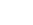functional requirements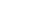Non – functional requirements

=38

To determine the specificity ( lack of ambiguity ) of requirements

A metric that is based on the consistency of the reviewers interpretation of each requirement.

Where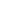is the number of requirements for which all reviewers had identical interpretations. The closer the value of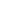to 1, the lower is the ambiguity of the specification.

The completeness of functional requirements can be determined by computing the ratio

Where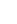is the number of unique function requirements,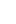is the number of inputs defined or implied by the specification andis the number of states specified.